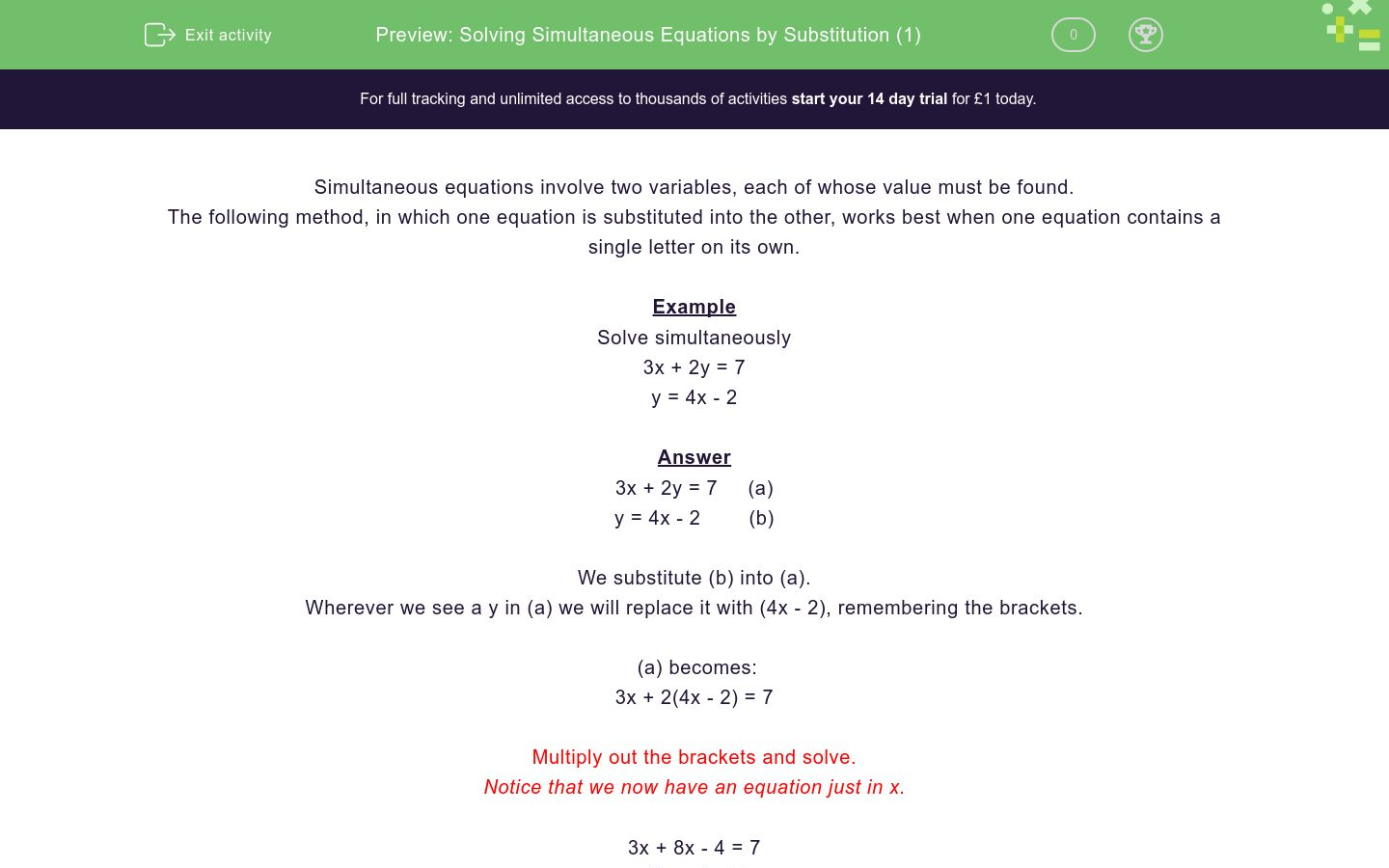# Solving Simultaneous Equations by Substitution (1)

In this worksheet, students solve simultaneous equations by substituting one equation into the other.Key stage:  KS 4

Curriculum topic:  Algebra

Curriculum subtopic:  Solve Simultaneous Equations in 2 Variables

Difficulty level:### QUESTION 1 of 10

Simultaneous equations involve two variables, each of whose value must be found.

The following method, in which one equation is substituted into the other, works best when one equation contains a single letter on its own.

Example

Solve simultaneously

3x + 2y = 7

y = 4x - 2

3x + 2y = 7     (a)

y = 4x - 2        (b)

We substitute (b) into (a).

Wherever we see a y in (a) we will replace it with (4x - 2), remembering the brackets.

(a) becomes:

3x + 2(4x - 2) = 7

Multiply out the brackets and solve.

Notice that we now have an equation just in x.

3x + 8x - 4 = 7

11x - 4 = 7

11x = 11

x = 1

Now use (b) to determine y.

y = 4x - 2

When x = 1, this gives:

y = 4×1 - 2 = 4 - 2 = 2

y = 2

Check by putting this into the other equation (a) to get 3 × 1 + 2 × 2 = 3 + 4 = 7

Solution is x = 1, y = 2

Solve simultaneously:

3x + 2y = 13

y = x - 1

x = 3, y = 2

x = 12, y = 3

x = 4, y = 1

Solve simultaneously:

x = y - 2

13x - 5y = 6

x = 6, y = -2

x = 2, y = 4

x = 4, y = 2

Solve simultaneously:

4x + 3y = 11

x = y + 1

x = 4, y = 2

x = 3, y = 2

x = 2, y = 1

Solve simultaneously:

2x + 5y = 31

y = 2x - 1

x = 4, y = -2

x = 13, y = 1

x = 3, y = 5

Solve simultaneously:

7x + 2y = 20

y = 4x - 5

x = 5, y = 5

x = 6, y = -11

x = 2, y = 3

Solve simultaneously:

5x + 6y = 17

y = 5x - 3

x = 1, y = 2

x = 3, y = 4

x = 2, y = 4

Solve simultaneously:

4x + 3y = 22

x = 2y

x = 10, y = -6

x = 6, y = 3

x = 4, y = 2

Solve simultaneously:

3x + 7y = 0

y = x - 10

x = 21, y = 1

x = 7, y = -3

x = 3, y = 7

Solve simultaneously:

5x + 3y = -33

y = -7 - x

x = -6, y = -1

x = 1, y = 4

x = 1, y = 3

Solve simultaneously:

6x + 5y = 29

x = y - 8

x = -1, y = 7

x = 1½, y = 4

x = 1, y = 10

• Question 1

Solve simultaneously:

3x + 2y = 13

y = x - 1

x = 3, y = 2
• Question 2

Solve simultaneously:

x = y - 2

13x - 5y = 6

x = 2, y = 4
• Question 3

Solve simultaneously:

4x + 3y = 11

x = y + 1

x = 2, y = 1
• Question 4

Solve simultaneously:

2x + 5y = 31

y = 2x - 1

x = 3, y = 5
• Question 5

Solve simultaneously:

7x + 2y = 20

y = 4x - 5

x = 2, y = 3
• Question 6

Solve simultaneously:

5x + 6y = 17

y = 5x - 3

x = 1, y = 2
• Question 7

Solve simultaneously:

4x + 3y = 22

x = 2y

x = 4, y = 2
• Question 8

Solve simultaneously:

3x + 7y = 0

y = x - 10

x = 7, y = -3
• Question 9

Solve simultaneously:

5x + 3y = -33

y = -7 - x

x = -6, y = -1
• Question 10

Solve simultaneously:

6x + 5y = 29

x = y - 8